# Hidden Markov Models Likelihood Computation – The Forward Algorithm

## Computing Likelihood

Given an Hidden Markov Model defined by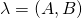and an observation sequence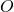, determine the likelihood that the observation sequence was generated by this model,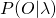.

For a particular hidden state sequence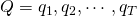and an observation sequence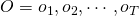, the likelihood of the observation sequence is defined as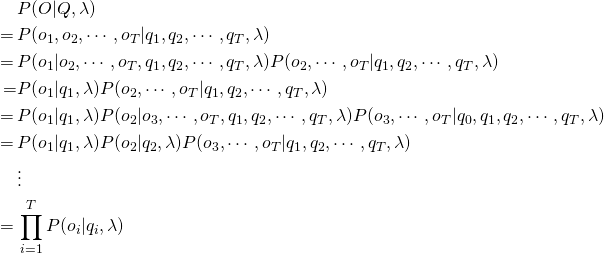The likelihood of the state sequence is defined as follows. The start state is excluded for now and added at the end.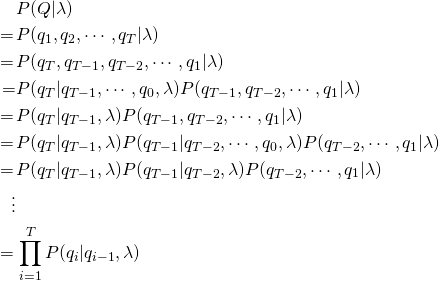where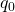is the same as the start state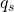.

Combining the two results above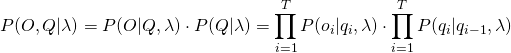The total probability of the observations can be computed by summing over all possible hidden state sequences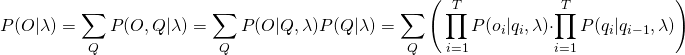## Time Complexity

A sequencecan have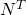combinations.

Thus the total time complexity will be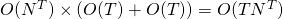.

## Forward Algorithm

The forward algorithm (a kind of dynamic programming algorithm) is an efficient algorithm, that uses a table to store intermediate values as it builds up the probability of the observation sequence. The forward algorithm computes the observation probability by summing over the probabilities of all possible hidden state paths that could generate the observation sequence, but it does so efficiently by implicitly folding each of these paths into a single forward trellis.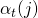represents the probability of being in state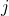after seeing the firstobservations, given the Hidden Markov Model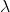.is computed by summing over the probabilities of every path that could lead to this state.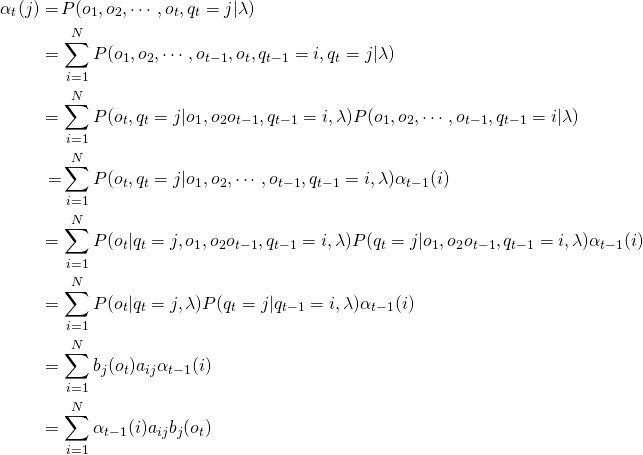where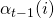is the previous forward path probability from the previous time step.

## Visualization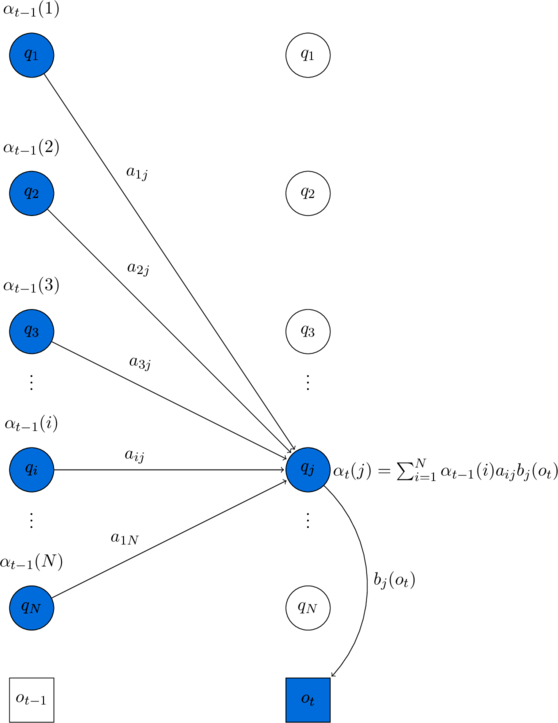Visualizing the computation of a single elementin the trellis by summing all the previous values, weighted by their transition probabilities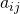, and multiplying by the observation probability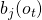. Hidden states are in circles, observations in squares. Shaded nodes are included in the probability computation for.

## Initialization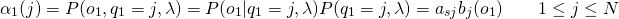## Recursion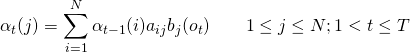## Termination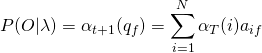## Time Complexity

Initialization step runs in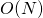. Recursion step is run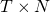times and the summation is over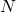. Thus, the recursion step runs in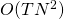. Termination step runs in. The forward algorithm thus reduces the time complexity to.